# Area

The term Area plays a vital role in contemporary mathematics. The area is the quantity that shows the amount of space occupied by a two-dimensional figure or shape or planar lamina, in the plane. In simple words, the area can be defined as the measure (the amount) of cloth or material with a thickness provided that would be required to construct a model of the shape or the necessary amount of paint to cover the surface of the shape with a single layer, i.e. one coat. It is the two-dimensional representation of the length of a curve or the volume of a solid, where length is a one-dimensional concept and the volume is a three-dimensional related concept.

## Area of Shapes

In geometry, the area of different shapes can be measured by comparing the shape to squares, which have a fixed size. We can find the area of simple and complex shapes using certain specific methods.

### 1. Area of Simple Shapes

In geometry, the area of shapes like quadrilaterals, circle that means 2d shapes can be found by counting the number of squares on a sheet of paper with grids (square shaped) and using simple formulas. These are explained in detail in the below sections, along with the necessary examples.

#### How to Find Area by Counting Squares?

Let us see an example to understand how to find the area of a particular shape by counting the number of squares.

Example: Find the area of shapes given in the figure.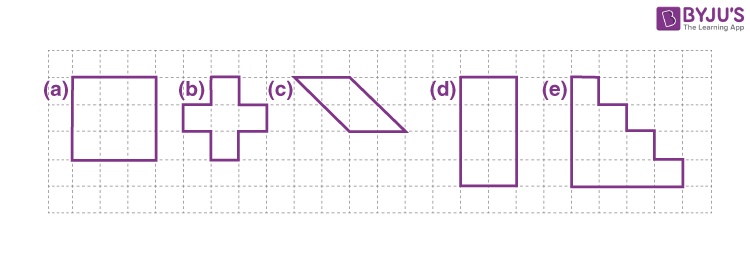Solution:

(a) Number of completely filled squares in the figure (a) = 9

Area covered by the shape (a) = 9 x 1 = 9 sq.units

(b) Number of completely filled squares in the figure (b) = 5

Area covered by the shape (b) = 5 x 1 = 5 sq.units

(c) Number of completely filled squares in the figure (c) = 2

Number of half filled squares in this figure = 4

Area of the shape (c) = 2 × 1 + 4 × (½)

= 2 + 2

= 4 sq.units

(d) Number of completely filled squares in the fourth figure (d) = 8

Area of the shape (d) = 8 x 1 = 8 sq.units

(e) Number of completely filled squares in the last figure (e) = 10

Area of the shape (e) = 10 x 1 = 10 sq.units

From this example we can derive the following rules for counting the number of squares to find the area of a shape. These are listed below:

• One full square with complete fill is taken as 1 square
• More than half of the square with filling is taken as 1 square
• Less than half of the square with filling is taken as 0, i.e. will not be taken for count

However, the area of shape obtained through this method is not accurate at every time. Hence, this is considered as the approximate area of the shape.

### 2. Area of Complex Shapes

Area of complex shapes can be found by splitting them into a group of similar simple shapes such as triangles, squares, rectangle and so on. The complex shapes are those shapes whose boundary is defined by joining several line segments.

For example, the area of the figure below can be found by making it into a group of triangles.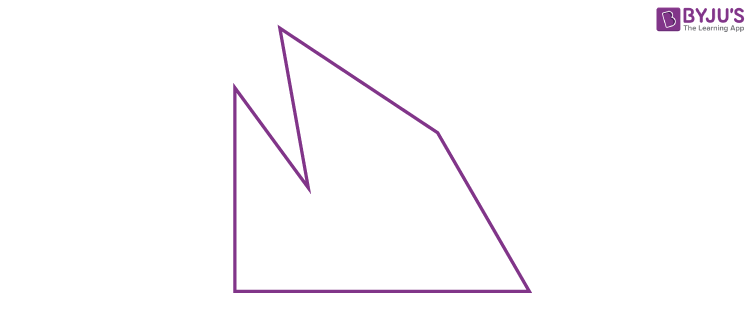This can be divided into triangles as: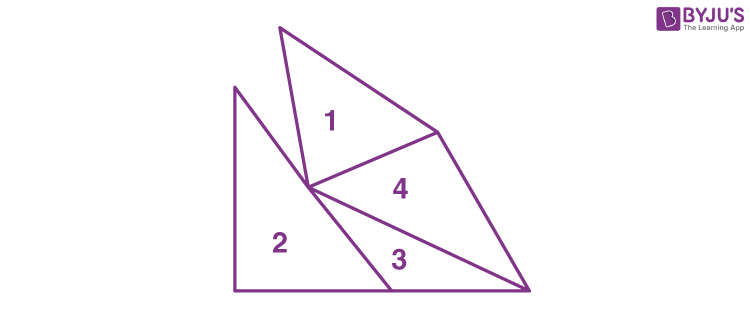Area of Two-Dimensional Shapes

The area of a two-dimensional figure is defined as the amount of space covered by the shape in a two-dimensional plane.

## Area Formulas

The formulas to find the area of different two dimensional figures are given below.

 Name Shape Area Parameters Circle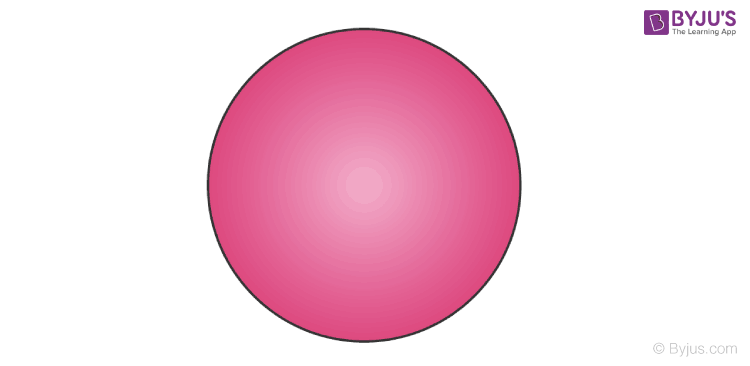πr2 r = Radius of the circle Triangle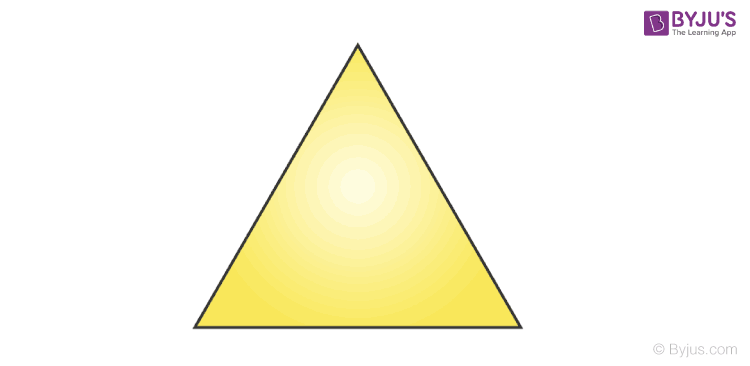(½)bh b = Base h = Height Square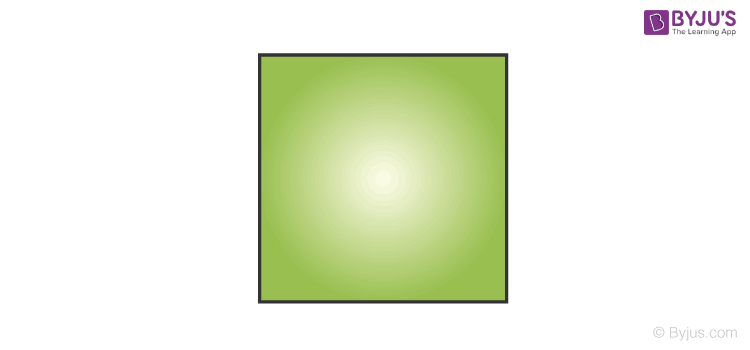a2 a = Length of the side Rectangle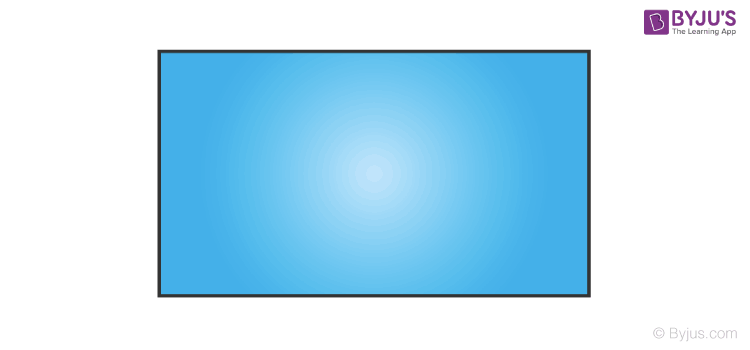lb l = Length b = Breadth Rhombus(½)d1d2 d1 and d2 are the lengths of two diagonals Parallelogrambh b = Base h = Height (distance between two parallel bases)

### Area Unit

All the units of length have a corresponding unit of area, for instance the area of a square concerning the given side length. Thus, areas can be measured in square millimetres (mm2), square centimetres (cm2), square metres (m2), square kilometres (km2), square feet (ft2), square yards (yd2), square miles (mi2), and so on. Algebraically, the units of an area can be considered as the squares of the corresponding length units.

One of the important properties of the area that are common to all the shapes in geometry is that; the value of area is always greater than or equal to zero, i.e. the measure of area will not be negative in any instance.

### Area Formula in Coordinate Geometry

In coordinate geometry, the area of two-dimensional shapes such as triangles and parallelograms can be found using the formulas. Here, the formula considers the coordinates of vertices of shape instead of length of the sides. The area of triangle with vertices (x1, y1), (x2, y2) and (x3, y3) is:

A = ½ [x1(y2 – y3) + x2(y3 – y1) + x3(y1 – y2)]

## Frequently Asked Questions on Area – FAQs

### How would you find the area?

To find the area of any shape, we must know the length or measures of required elements to substitute in the respective formulas. For example, to find the area of a square, we need the length of one of the sides.

### What is the formula for the area of all shapes?

The formulas to find the areas of few shapes are given below:
Area of circle = πr^2, where r is the radius of the circle.
Area of triangle = (1/2)bh, where b is the base and h is the triangle’s height.
Area of square = a^2, where a is the side of a square.
Area of rectangle = lb, where l is the length and b is the rectangle’s breadth.

### What is the area of a shape?

The area of a shape is the space enclosed within the boundary or perimeter of the given shape. Different math formulas are available to find the areas of different shapes.

### What is area and perimeter in maths?

In maths, the perimeter is the distance around the outside of a shape, and the area measures the space inside a shape, i.e. the space enclosed by the boundary of that shape.

### What are perimeter and area examples?

For example, when we want to fence the garden at our house, the fencing material’s length is the perimeter of the garden. The area is the space, i.e. the total space of that garden in the specified example.

### How do you find the area and perimeter?

To find the area of any shape, we have to find the number of square units of space occupied by the shape. To find the perimeter of the shape, we have to find the length of the boundary of that shape.